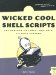24 An Interactive Calculator

#24 An Interactive Calculator

Once I wrote Script #9, allowing command-line invocations of bc for floatingpoint calculations, it was inevitable that I'd write a small wrapper script to create an interactive command- line-based calculator. What's remarkable is that, even with help information, it's very short.

The Code

#!/bin/sh # calc - A command-line calculator that acts as a front end to bc. scale=2 show_help() { cat << EOF   In addition to standard math functions, calc also supports   a % b       remainder of a/b   a ^ b       exponential: a raised to the b power   s(x)        sine of x, x in radians   c(x)        cosine of x, x in radians   a(x)        arctangent of x, returns radians   l(x)        natural log of x   e(x)        exponential log of raising e to the x   j(n,x)      bessel function of integer order n of x   scale N     show N fractional digits (default = 2) EOF } if [ \$# -gt 0 ] ; then   exec scriptbc "\$@" fi echo "Calc - a simple calculator. Enter 'help' for help, 'quit' to quit." echo -n "calc> " while read command args do   case \$command   in     quitexit) exit 0                                  ;;     help\?)   show_help                               ;;     scale)     scale=\$args                             ;;     *)         scriptbc -p \$scale "\$command" "\$args"   ;;   esac   echo -n "calc> " done echo "" exit 0

How It Works

There's really remarkably little of a complex nature going on here. Perhaps the most interesting part of the code is the while read statement, which creates an infinite loop that displays the calc> prompt until the user exits, either by typing quit or entering an end-of-file sequence ( ^D ). And, of course, the simplicity of this script is exactly what makes it wonderful: Shell scripts don't need to be extremely complex to be useful!

Running the Script

This script is easily run because by default it's an interactive tool that prompts the user for the desired actions. If it is invoked with arguments, those arguments are passed to the scriptbc command instead.

The Results

\$  calc 150 / 3.5  42.85 \$  calc  Calc - a simple calculator. Enter 'help' for help, 'quit' to quit. calc>  help  In addition to standard math functions, calc also supports   a % b       remainder of a/b   a ^ b       exponential: a raised to the b power   s(x)        sine of x, x in radians   c(x)        cosine of x, x in radians   a(x)        arctangent of x, returns radians   l(x)        natural log of x   e(x)        exponential log of raising e to the x   j(n,x)      bessel function of integer order n of x   scale N     show N fractional digits (default = 2) calc>  54354 ^ 3  160581137553864 calc>  quit  \$Wicked Cool Shell Scripts
ISBN: 1593270127
EAN: 2147483647
Year: 2004
Pages: 150
Authors: Dave Taylor

Similar book on Amazon